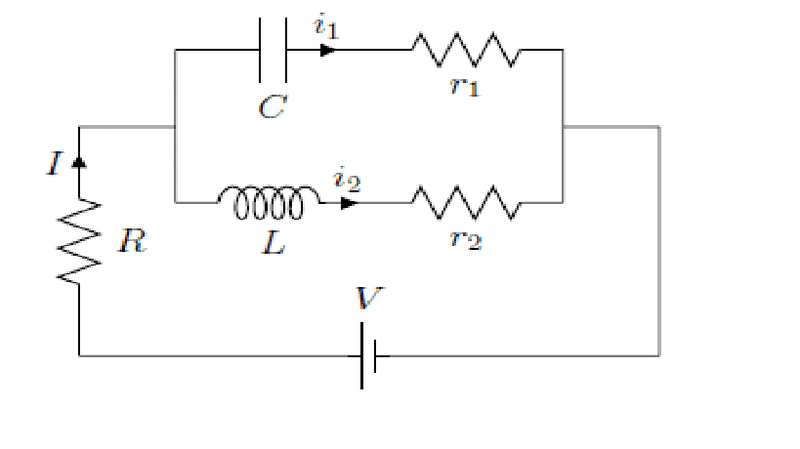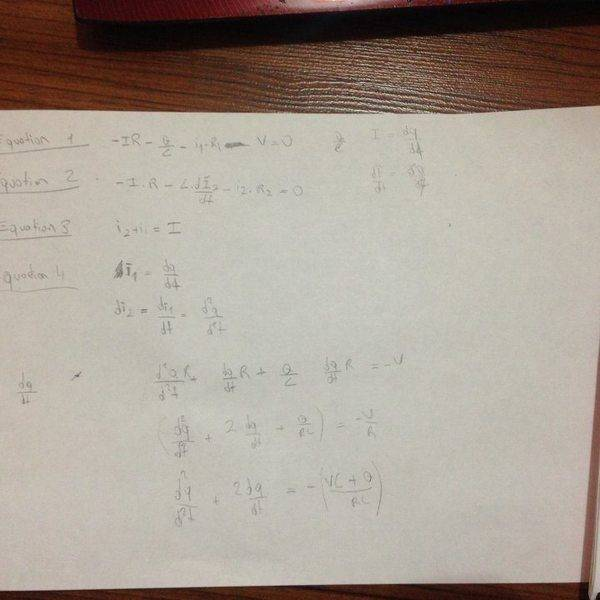# RLC Circuit Question For final exam

• Hapusss

## Homework Statement

[/B]
(a) Write down sucient number of equations to determine all the
currents i1, i2 and I (you do not need to solve the equations)
(b) At the instant the battery is turned on, what is the current I
running through the circuit?
(c) After waiting for a suciently long time after the battery is turned
on, what is the current I running through the circuit?## Homework Equations

i get 4 equation. You can get them down of the page

## The Attempt at a Solution

To start, I pick 2 ways and i tried kirchhoff rule and for current i1+i2=I. dq/dt=i1 these are my prediction. İf u have a look my work upload at down, u ll understand my solution attempt.[/B]

Last edited:
Hi Hap,Not a very good start. What does the relevant equation stand for ?
How about checking with your notes or textbook to find what equation describes a capacitor ? Idem inductance ?

Did you check the PF guidelines ? 'Don't know where to start' kind of isn't good enough...

I tried to get quick response but it didnt work so i tried somethings. Here is some works but i can't get question b and c. Maybe i can get little bit help from you guys.

For 2nd equation: there should be mines V.